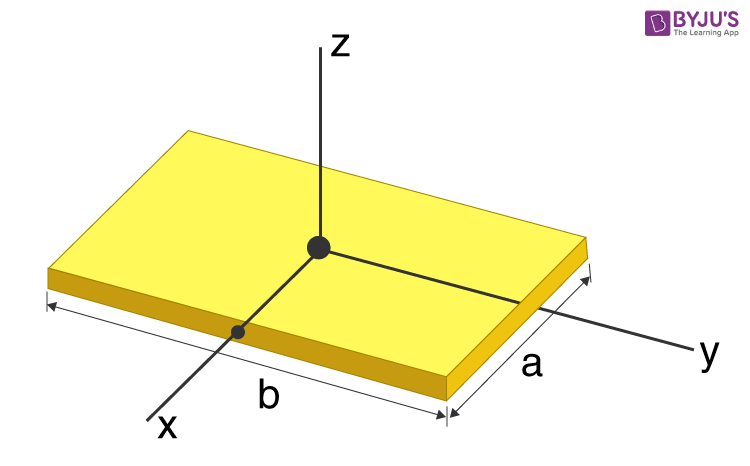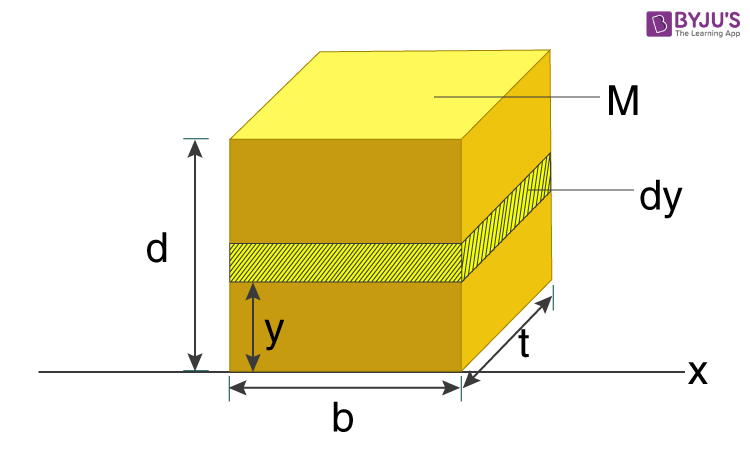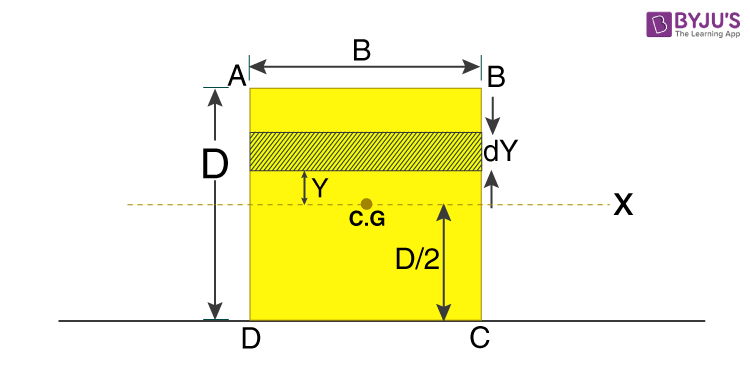Checkout JEE MAINS 2022 Question Paper Analysis : Checkout JEE MAINS 2022 Question Paper Analysis :

# Moment Of Inertia Of A Rectangular Plate

In the case of a rectangular plate, we usually find the mass moment of inertia when the axis is passing through the centre perpendicular to the plane.We use the following expressions to calculate the moment of inertia of a rectangular plate;

For x-axis;

 Ix = (1/12) mb2

For y-axis;

 Ix = (1/12) ma2

And,

 IZ = (1/12) m (a2 + b2)

## Moment Of Inertia Of A Rectangular Plate Derivation

### 1. Line Passing Through The BaseFor the derivation of the moment of inertia formula for a rectangular plate, we will consider a rectangular section and cut out an elemental part at a distance (y) from the x-axis. Let its thickness be dy and s be the mass per unit volume of the plate.

Mass of the rectangular body = density x volume

M = ρ x bdt

Mass of the elemental section;

dM =ρ  x btdy

Now we will find the mass moment of inertia of the elemental strip about the x-axis.

I = ∫ y2 dM

I = ∫ (ρ b dy t)y2

In the next step, we will find the mass moment of inertia of the rectangular plate.

I = od ρ b dy t y2

I = ρ bt od y2dy

I = ρ bt d3 / 3

(since ρ  = M/bdt)

I = Md2 / 3

At this point, we apply the parallel axis theorem which gives us;

Ibase = ICG + M x h2

ICG = Ibase – Mh2

ICG = Md2 / 3 – M x (d / 2)2

ICG = Md2 / 12

This is how we determine the mass moment of inertia of a rectangular section about a line passing through the base.

### 2. Line Passing Through The Centre of Gravity

Now if we want to derive the expression for a line passing through the centre of gravity then the steps for derivation are almost the same. We only have to make a few changes.We will take one rectangular elementary strip and consider the thickness to be (dY) and it will be at a distance (Y) from the X-X axis.

The rectangular elementary strip area will be dA = dY.B

Mass of the rectangular elemental part is given by;

dm = ρ x T x dA

dm = ρ x T x dY. B

dm =ρBT. dY

Now we can say that the mass moment of inertia of the rectangular elemental section about the X-X axis ,

(Im)xx = dm.Y2

If we substitute the values for dm we get;

(Im)xx = ρBT. Y2dY

Next step involves determining the mass moment of inertia of the entire rectangular section. We have to integrate the above equation between limit (-D/2) to (D/2). We will write it as;

(Im)xx = -D/2D/2 ρBT. Y2dY

(Im)xx = ρBT -D/2D/2 Y2dY

(Im)xx = ρBT [y3 / 3]-D/2D/2

(Im)xx = ρBT / 3 [(D/2)3 – (-D/2)3]

Im) XX = ρBT.D3/12

(Im) XX = ρBTD.D2/12

(Im) XX = ρV.D2/12

(Im) XX = M.D2/12

Likewise, we can also determine the mass moment of inertia of the rectangular section about the Y-Y axis. We will obtain;

(Im) YY = M.B2/12

## Video lesson – Parallel Axis Theorem